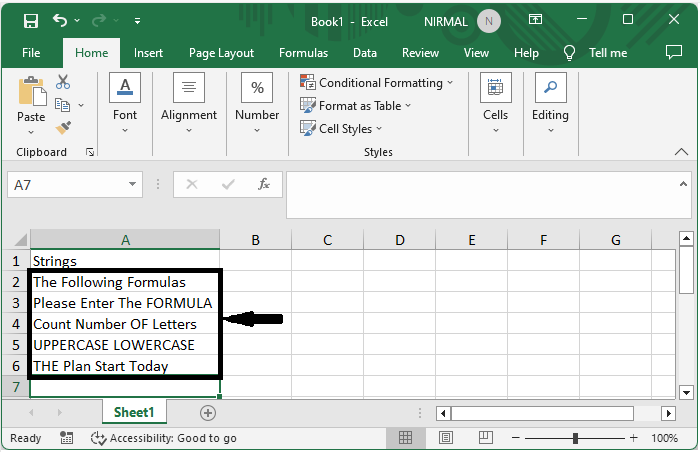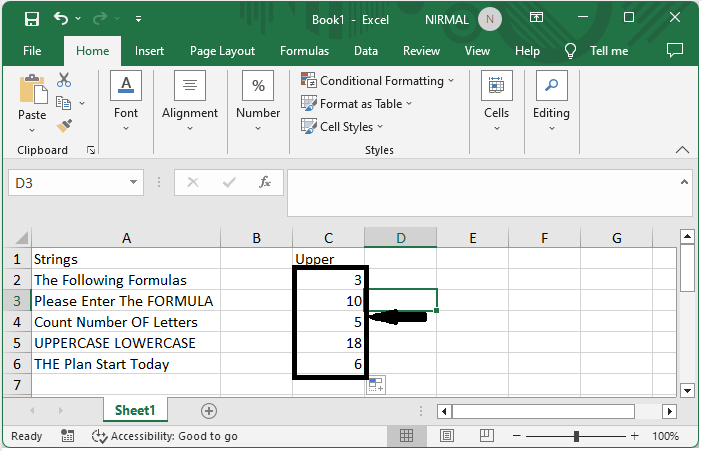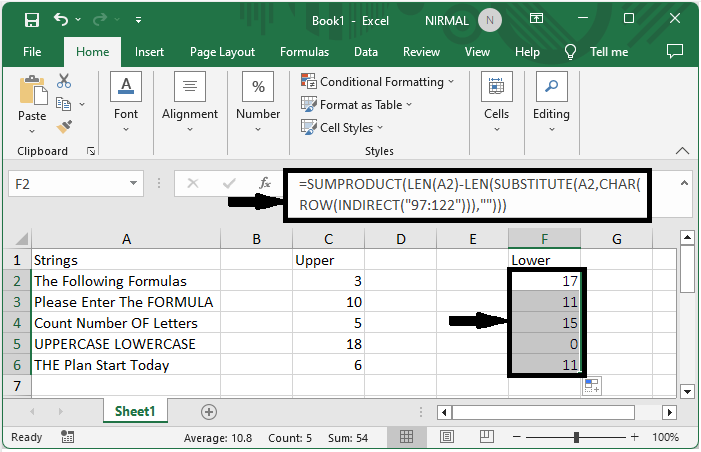# How to Count the Number of Uppercase or Lowercase Letter in Cell?

Excel is a robust programme that enables you to carry out numerous calculations and data manipulations. Extracting specific information from the text contained in cells through analysis is a frequent activity. This lesson is for you if you need to count the amount of uppercase or lowercase characters in a cell when dealing with text data in Excel.

In this lesson, we'll show you how to use Excel's built-in functions and formulae to count the amount of uppercase or lowercase letters in a cell. Regardless of your level of Excel proficiency, this tutorial will give you the knowledge and abilities you need to do this work successfully.

## Count the Number of Uppercase or Lowercase Letter in Cell

Here we can count the number of uppercase and lowercase letters using the formula. So let us see a simple process to learn how you can count the number of uppercase or lowercase letters in a cell in Excel.

### Step 1

Consider an Excel sheet where you have a list of strings with lowercase and uppercase characters, similar to the below image.First, to count the number of uppercase letters, click on an empty cell and enter the formula =SUMPRODUCT(LEN(A2)-LEN(SUBSTITUTE(A2,CHAR(ROW(INDIRECT("65:90"))),""))) and click enter to get the first value. Then drag down using the autofill handle.

Empty cell > Formula > Enter > Drag.### Step 2

Now to count the number of uppercase letters, click on an empty cell and enter the formula as =SUMPRODUCT(LEN(A2)-LEN(SUBSTITUTE(A2,CHAR(ROW(INDIRECT("65:90"))),""))) and click enter, then drag down using the auto fill handle.

Empty cell > Formula > Enter > Drag.This is how you can count the number of uppercase and lowercase letters in a cell in Excel.

## Conclusion

In this tutorial, we have used a simple example to demonstrate how you can count the number of uppercase or lowercase letters in a cell in Excel to highlight a particular set of data.

Updated on: 22-Aug-2023

183 Views